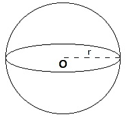# Sphere Formula

## Sphere Formulas - Surface Area of a Sphere Formula

Formulas related to Spheres
A sphere is a 3D object which can be defined as the collection of all the points in space that are equidistant from a given fixed point known as the centre. The same definition when applied to a 2D Euclidean plane results in a circle. So, the sphere may be thought of as the 3D version of a circle. Like a circle, it has a fixed centre and all the points on its surface (3D equivalent of circumference) are at a fixed distance from the centre known as the radius. The following diagram shows a general sphere of radius r units with centre O.Some of the important formulas related to spheres are listed below.
Diameter, d = 2r
Surface area, $SA = 4\pi {r^2}$
Volume, $V = \frac{{4\pi }}{3}{r^3}$
Let’s look at an example to see how to use these formulas!

Question: Find the surface area of a sphere whose volume is 38808 cm3.
Solution:
$V = \frac{{4\pi }}{3}{r^3} = 38808 \Rightarrow \frac{4}{3} \times \frac{{22}}{7} \times {r^3} = 38808 \Rightarrow {r^3} = \frac{{38808 \times 21}}{{88}} = 9261 \Rightarrow r = 21\,\,cm$
$SA = 4\pi {r^2} = 4 \times \frac{{22}}{7} \times {21^2} = 4 \times 22 \times 3 \times 21 = 5544\,\,c{m^2}$
Why don’t you try to solving a problem for practice?

Question: The surface area of a sphere is 616 cm2. Find its volume.
Options:
(a) $143.34\,c{m^3}$
(b) $616.67\,c{m^3}$
(c) $437.34\,c{m^3}$
(d) $1437.34\,c{m^3}$
$SA = 616\,c{m^2}$
$SA = 4\pi {r^2}\, \Rightarrow 4\pi {r^2} = 616 \Rightarrow {r^2} = \frac{{616 \times 7}}{{4 \times 22}} = 49 \Rightarrow r = 7\,cm$
$V = \frac{{4\pi }}{3}{r^3} = \frac{4}{3} \times \frac{{22}}{7} \times {7^3} = 1437.34\,c{m^3}$